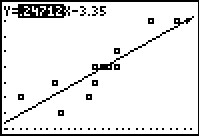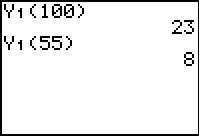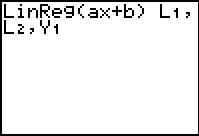# Activities

••• ##### Subject Area

• Math: Algebra I: Data Analysis
• Math: Algebra I: Linear Functions

• ##### Author9-12

15 Minutes

• ##### Device
• TI-83 Plus Family
• TI-84 Plus
• TI-84 Plus Silver Edition
•TI-84 Plus C Silver Edition
•TI-84 Plus CE
• ##### Software

TI Connect™
TI Connect™ CE

• ##### Accessories

TI Connectivity Cable

• ##### Report an Issue

Chirp, Jump, Scatter#### Activity Overview

Students will find a best fit line for data graphed as scatter plots.

#### Key Steps

•Students will run a program that contains the data sets for each problem. When they select an option, it will enter the lists of data into L1 and L2. Students are to look at the lists and determine if they can see a trend.

Students will create a scatter plot and then use the Line command to create a line of best fit.

•Then students will use the two points of their line to calculate the equation. Using the equation, students will predict the number of chirps for a given temperature.

•Students can compare their line of best fit with the calculator’s linear regression line by adding both lines.